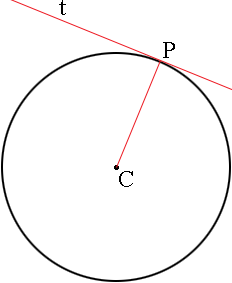SEARCH HOMEMath Central Quandaries & QueriesQuestion from debz, a student: what is the formulae of the tangent to a circle.... our teacher gave us a lot of homework.. and she ask us to find the formulae by ourselves..Debz,

I think that waht your teacher is looking for is tha fact that if $C$ is the center of a circle, $P$ is a point on the circle and $t$ is the tangent line to the circle at $P$ the the radius $CP$ is perpendicular to $t.$PennyMath Central is supported by the University of Regina and The Pacific Institute for the Mathematical Sciences.